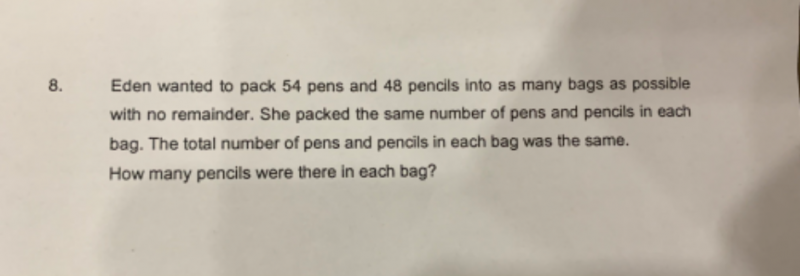# Questionfactors of 54(pens)     factors of 48(pencils)

1 x 54                                     1 x 48

2 x 27                                     2 x 24

3 x 18                                     3 x 16

6 x 9                                       4 x 12

6 x 8

So into as many bags as possible would be 6 bags.

Hence the number of pencils in each bag is 8.

2 Replies 2 Likes ✔Accepted Answer

You are so right!

They are using the word “same” in different ways to confuse the students.  In my opinion, Math- English should be precise whereas English-English (haha) can be ambiguous but they are using English-English here and it confuses a lot….. so I wonder if they are learning Math or English. lol

“She packed the same number of pens and pencils in each bag”….this “same” refers to…for every bag, the same number of pens, the same number of pencils are packed but it does not mean they are equal number for pens and pencils.    Eg… 3 pens in every bag and 4 pencils in every bag….

“The total number of pens and pencils in each bag was the same”…this “same” refers to equal.  That means if total is 7 for one bag, every bag is 7.    This sentence could have been worded as “The total number of pens and pencils in each bag was equal.”     This is more precise Math-English and make the students think of the differences in the two statements.

To summarise in Math lingo which is so easy to understand…lol

same <> equal   but

equal = same

0 Replies 1 Like

Thank you Sushi88!